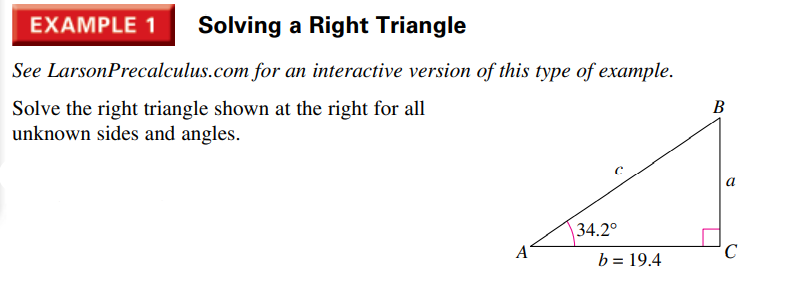### Still have math questions?

Trigonometry
QuestionSee LarsonPrecalculus.com for an interactive version of this type of example. Solve the right triangle shown at the right for all unknown sides and angles.

$$a = 13.18423$$
$$c = 23.456$$
$$\angle \beta = 55.8 ^ { \circ } = 55 ^ { \circ } 48 ^ { \prime } 0 ^ { \prime \prime }$$